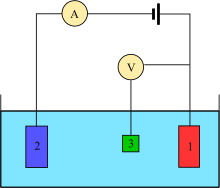## How to Calculate and Solve for Electrode Potential | Nernst Equation | CorrosionThe image above represents electrode potential.

To compute for electrode potential, three essential parameters are needed and these parameters are Standard Electrode Potential (Eo), Number of Electrons (n) and Molar Activity (Cion).

The formula for calculating electrode potential:

E = Eo0.059/nIn(Cion)

Where:

E = Electrode Potential | Nernst Equation
Eo = Standard Electrode Potential
n = Number of Electrons
Cion = Molar Activity

Let’s solve an example;
Find the electrode potential when the standard electrode potential is 14, the number of electrons is 10 and the molar activity is 12.

This implies that;

Eo = Standard Electrode Potential = 14
n = Number of Electrons = 10
Cion = Molar Activity = 12

E = Eo0.059/nIn(Cion)
E = 14 – 0.059/10In(12)
E = 14 – (0.0059)(2.48)
E = 14 – 0.0146
E = 13.98

Therefore, the electrode potential is 13.98 V.